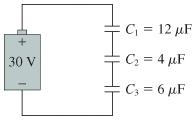# Problem: A. What is the charge on each capacitor in the figure? Enter your answers numerically separated by commas.B. What is the potential difference across each capacitor in the figure? Enter your answers numerically separated by commas.

###### FREE Expert Solution

For capacitors in series, the equivalent capacitance is:

$\overline{)\frac{\mathbf{1}}{{\mathbf{C}}_{\mathbf{e}\mathbf{q}}}{\mathbf{=}}\frac{\mathbf{1}}{{\mathbf{C}}_{\mathbf{1}}}{\mathbf{+}}\frac{\mathbf{1}}{{\mathbf{C}}_{\mathbf{2}}}{\mathbf{+}}{\mathbf{.}}{\mathbf{.}}{\mathbf{.}}{\mathbf{+}}\frac{\mathbf{1}}{{\mathbf{C}}_{\mathbf{n}}}}$

A.

Ceq = (1/C1 + 1/C2 + 1/C3)-1 = (1/12 + 1/4 + 1/6)-1 = 2µF

85% (214 ratings)###### Problem DetailsA. What is the charge on each capacitor in the figure? Enter your answers numerically separated by commas.

B. What is the potential difference across each capacitor in the figure? Enter your answers numerically separated by commas.